## teaching and educationJOURNAL OFAPPLIEDCRYSTALLOGRAPHY
ISSN: 1600-5767
Volume 46| Part 1| February 2013| Pages 242-247

## The prolific polytypism of silicon carbide

*Correspondence e-mail: alortiz@materiales.unex.es

(Received 26 June 2012; accepted 29 November 2012; online 17 January 2013)

A worked example of polytypism is presented, aimed at assisting undergraduates in the learning and instructors in the teaching of this topic. In particular, this crystallography concept, not necessarily obvious for beginners, is illustrated pedagogically using to that end the model case of the prolific polytypism of silicon carbide (SiC). On the basis of concepts that are easily assimilated by students (i.e. simple topological constraints) this article first presents a unified description of the polytypism phenomenon in SiC that allows one to understand without difficulty the existence of its numerous polytypic variants and how they develop. Then the various notations used to designate these different polytypes are described, and finally the crystal structures of the most common are discussed. This worked example is thus expected to contribute to motivating undergraduates in the study of a crystallography topic that often is not treated in sufficient depth in class.

### 1. Introduction

A good knowledge of crystallography, the science devoted to crystal structures, is very important in the study of other disciplines such as physics, chemistry, geology and engineering, and is also relevant in others such as biology and mathematics, to name just a few. It is not surprising therefore that the study of crystallography is part of the undergraduate curricula of many academic degree programs at most universities around the world. In some of these programs, crystallography is a core subject, with separate, specific credits devoted to its learning. In the engineering degrees, however, crystallography typically does not constitute by itself an entire subject of study but is introduced to the undergraduate within broader courses on materials science. In this scenario, the learning of crystallography is sometimes difficult, or at least problematic, for two reasons. Firstly, crystallography taught as a lecture course is a very dry subject for undergraduates with a pure engineering background, as it involves the learning of concepts that are not necessarily obvious for beginners. Secondly, instructors do not have sufficient time to develop crystallographic concepts to the required depth in class because of the great diversity of content to be covered in a typical materials science course. Fortunately, most textbooks on materials science (e.g. Shackelford, 2009; Askeland et al., 2011; Callister & Rethwisch, 2012) devote various chapters to discussing in detail crystallographic concepts such as bonding, the Bravais lattice, the unit cell, crystal structure, defects in crystals and others, and to explaining them pedagogically by presenting illustrative examples and practical exercises together with the abstract concepts. Unfortunately, other concepts, such as for instance polymorphism and polytypism, are hardly ever treated adequately, despite their also being important for the correct understanding of other materials science subjects, such as phase transformations and properties in metals and ceramics. These concepts are of course covered appropriately in crystallography books and treatises (Verma & Krishna, 1966; Krishna & Verma, 1966; Trigunayat & Chadha, 1971; Trigunayat, 1991), but these texts are often too advanced for engineering students because crystallography is introduced at a relatively early stage in their degree course. Clearly, to motivate the study of these other crystallographic concepts it is even more vital than before to introduce them together with adequate worked examples that involve only simple concepts and that can arouse the interest of engineering students.

With these premises in mind, this teaching and education paper is aimed at presenting a worked example of polytypism, in particular a unified description of the polytypism in silicon carbide (SiC) that is at the same time appealing and useful. Since we use only concepts that are easily assimilated by students, in particular simple topological constraints, this example of polytypism can be either taught in class or left to be assimilated individually by the undergraduates as homework with relatively little effort. Discussion of the theories explaining the occurrence and stability of the different SiC polytypes is far beyond the purpose of the present paper.

### 2. Unified view of polytypism in SiC

SiC is the only compound that can be formed by combining silicon (Si) and carbon (C), as is shown in the Si–C phase diagram presented in Fig. 1(Olesinski & Abbaschian, 1984). Despite SiC being a very important advanced ceramic with major engineering applications (both structural and functional), our interest here in SiC is exclusively academic because of its prolific polytypism, which will serve as a good example to illustrate this crystallographic phenomenon to students just initiated into crystallography. For the present purposes, it suffices to define polytypism simply as the ability of a compound to exist in different crystallographic forms that differ essentially in one crystallographic direction, achieved by variations in the atomic layer stacking sequence (Baumhauer, 1912). What distinguishes SiC from other compounds with polytypism is its capacity to crystallize, somewhat unpredictably, in very many polytypic variants. Indeed, to date more than 250 different polytypes have been discovered, with repeats of the stacking sequence varying from just two layers to many hundreds of layers (Fisher & Barnes, 1990). In all the SiC crystal structures, however, the Si and C atoms are always in tetrahedral coordination, as expected for a three-dimensional covalently bonded compound of group IV elements with sp3 orbital hybridization. As shown in Fig. 2, with this configuration each C atom is located at the centroid of a tetrahedron at whose vertices there are Si atoms, and vice versa. Evidently, this geometry leads to the fourfold coordination [Si4C in Fig. 2(a), and C4Si in Fig. 2(b)] that is the signature of covalently bound structures.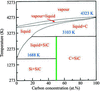Figure 1 The Si–C phase diagram. The thick vertical line marks SiC. Adapted from Olesinski & Abbaschian (1984).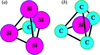Figure 2 Simple tetrahedral coordination of Si and C atoms: (a) Si4C tetrahedron (C atom located at the tetrahedron's centroid and Si atoms at its vertices) and (b) C4Si tetrahedron (Si atom located at the tetrahedron's centroid and C atoms at its vertices).

From Fig. 2it is clear that the tetrahedral coordination of the atoms (C or Si) located at the centroid of the tetrahedron is always ensured by the presence of atoms of the other chemical species (Si or C) at the four vertices of the tetrahedron. Hence, it is the constraint of the tetrahedral coordination of the atoms located at these vertices that imposes certain restrictions on how the tetrahedra themselves are linked and stacked. Shown in Fig. 3are the only two possibilities that satisfy the double tetrahedral coordination, which, as can be observed, themselves differ at first sight in the relative orientation between the upper and lower tetrahedra [they either have the same orientation, as seen in Fig. 3(a), or are rotated 180° with respect to each other, as seen in Fig. 3(b)]. Hereafter, to distinguish between these two possibilities, the unrotated tetrahedra (also often called untwinned tetrahedra) will be denoted as tetrahedra of type T and the rotated ones (also called twinned tetrahedra) as tetrahedra of type T′. As can be observed in Fig. 3, the tetrahedra have to be stacked in layers in such a way that the triangular bases defined by their lower three vertices define a plane. These vertices are shared by three tetrahedra belonging to the same layer, and also by one of the tetrahedra located in the layer immediately below. Clearly, the top vertices of the tetrahedra of a given layer in turn generate the lower vertices of the tetrahedra of the layer located immediately above. Furthermore, each vertex is shared by four different tetrahedra whose centroids in turn give rise to an inverted tetrahedron with its centroid at the common vertex, and in addition, two neighbouring tetrahedra share one and only one of their four vertices.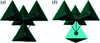Figure 3 Double tetrahedral coordination of Si (in grey) and C (in black) atoms: (a) two tetrahedron layers with the same relative orientation and (b) two tetrahedron layers with a relative rotation of 180°.

In order to explain why only these two arrangements are possible, it is very useful didactically to analyse the packing in the plane and the stacking in the space of the tetrahedra (Si4C or C4Si), utilizing to that end two-dimensional projections. To distinguish between tetrahedron layers and simple tetrahedra, the former will hereafter be denoted using boldface letters in the form T or T′, again with the use of the prime representing the 180° rotation. Let us denote by T1 the first layer of tetrahedra whose plane projection is shown in Fig. 4(a), where the subscript 1 indicates the plane projection of the positions of the centroids and top vertices of the tetrahedra. As can be easily visualized, there exist only two possible manners of stacking the next tetrahedron layer upon this one, if the condition is imposed that the bottom vertices of these new tetrahedra have all to be placed in positions 1 in order to satisfy the constraint that the top vertices of a tetrahedron layer have to generate the bottom vertices of the next layer. These possibilities are (i) stacking of a tetrahedron layer with the same orientation as before in T1, but this time with the tetrahedron centroids and top vertices located in the positions labelled 2 as shown in Fig. 4(b), so that this new tetrahedron layer will be denoted T2; and (ii) stacking of a layer of tetrahedra rotated 180° relative to the tetrahedra in layer T1, and in addition with the tetrahedron centroids and top vertices located at the positions labelled 3 as shown in Fig. 4(c), so that this new tetrahedron layer will be denoted T3′. If this pattern continues with the stacking of new tetrahedron layers, it is easy to deduce that both the tetrahedra T and T′ can only take the positions labelled as 1, 2 and 3 in Fig. 5. Therefore, one concludes that there are a total of only six possible types of tetrahedron layers (Ti or Ti′, with i = 1, 2 or 3; the numerical labels 1, 2 and 3 can be changed to the letters A, B and C if preferred). It is worth mentioning that the hexagonal arrangement of the positions of the tetrahedron centroids labelled 1, 2 and 3 (see for example Fig. 4a) is reminiscent of the stacking of idealized hard spheres used typically to illustrate the construction of the hexagonal and cubic close-packed structures, although in these latter cases there are only three possible types of sphere layers (denoted typically as A, B and C) owing to the invariance of spheres with respect to rotations.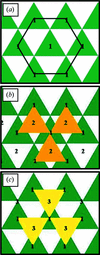Figure 4 Two-dimensional representation of the spatial distribution of tetrahedra: (a) positioning of the first tetrahedron layer, (b) stacking of a new tetrahedron layer with the same relative orientation as before and (c) stacking of a new tetrahedron layer rotated by 180°.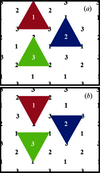Figure 5 The six possible manners of placing the tetrahedron layers: (a) untwinned tetrahedra situated in positions 1, 2 or 3, and (b) twinned tetrahedra also in positions 1, 2 or 3.

Clearly, each one of the numerous polytypic variants of SiC has its own characteristic stacking sequence, formed by combining some or all of these six possible types of tetrahedron layers. However, it is important to mention that a key aspect is that these stacking sequences are not random, but they have necessarily to satisfy certain constraints. This is because on a given tetrahedron layer it is only possible to stack two of the five remaining tetrahedron layers, one of which will be formed by tetrahedra with the same orientation as before and the other by tetrahedra rotated 180°. In particular, Fig. 6shows that on type T1 and T1′ layers, only type T2 or T3′ layers can be stacked; on type T2 and T2′ layers, only type T3 or T1′ layers can be stacked; and on type T3 and T3′ layers, only type T1 or T2′ layers can be stacked. Note that, because the top vertices of the tetrahedra of the layers T and T′ with the same subscript occupy the same positions, the tetrahedron layers that can be stacked on them are necessarily the same.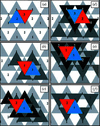Figure 6 The two possible tetrahedron layers that can be stacked on a given layer, always one with the same relative orientation and the other rotated by 180°: (a) T2 or T3′ on T1, (b) T3 or T1′ on T2, (c) T1 or T2′ on T3, (d) T2 or T3′ on T1′, (e) T3 or T1′ on T2′, and (f) T1 or T2′ on T3′.

According to the above discussion, it can be deduced that the different stacking sequences have to satisfy certain constraints, which can be expressed mathematically as follows:

(1) A given tetrahedron layer of the type T or T′ is followed by another tetrahedron layer with the same relative orientation (T or T′) according to the following rules: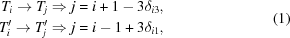where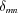is the Kronecker delta function (which adopts the value 1 if m = n and 0 if mn).

(2) A given tetrahedron layer of the type T or T′ is followed by another tetrahedron layer rotated 180° (T′ or T) according to the following rules: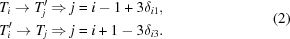These mathematical restraints can be more easily visualized in the flow chart shown in Fig. 7. As can be seen, it consists of a set of two concentric equilateral triangles whose vertices are occupied only by tetrahedron layers of the type T or T′, and the arrows that emanate from each tetrahedron layer point to the two unique possible subsequent tetrahedron layers. Logically, movements within each of these two triangles correspond to stacking tetrahedron layers of the same type (T or T′), whereas jumps from one triangle to the other generate orientation changes of the tetrahedron layers.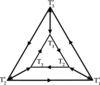Figure 7 Flow chart of the stacking sequence of tetrahedron layers in the SiC polytypes.

Finally, it is worthwhile to mention that in principle one could generate an infinite number of possible stacking sequences that satisfy the above restraints, and therefore, theoretically, the number of potential SiC polytypes is infinite. This is entirely consistent with the great number of different SiC polytypes discovered to date.

### 3. Naming the different SiC polytypes

Originally, the different SiC polytypes were denoted by labels in the order that they were being discovered, using simply the succession of Roman numerals I, II, III and so on. However, this notation soon became impractical as the number of discovered polytypes increased dramatically, which made it necessary to use some other nomenclature. Evidently, one possibility would be to list the entire sequence of tetrahedron layers T and T′. However, while this notation is probably a complete and unambiguous representation of the polytypes, in practice it is very tedious as it is not at all compact, especially for those polytypes with long stacking sequences. Thus, today there exist various other notations to denote and more easily differentiate the huge number of existing and future' SiC polytypes. The most common of these notations is the Ramsdell (1945) notation. It does not distinguish between untwinned and twinned tetrahedron layers, and designates the polytypes merely as nX, where n is an integer number that gives the order of periodicity (i.e. the total number of tetrahedron layers in the stacking sequence) and X is a letter that indicates the type of Bravais lattice (C for cubic, H for hexagonal and R for rhombohedral). Given the lack of distinction between untwinned and twinned tetrahedron layers in the Ramsdell notation, the layers T1 and T1′ are often both denoted simply as layer A, T2 and T2′ as layer B, and T3 and T3′ as layer C, which constitutes the so-called ABC notation.

Another two designations that essentially derive from the ABC notation are the Hägg (1943) notation and the Nabarro–Frank notation (Frank, 1951). In the Hägg notation, the cyclic movements AB, BC and CA are all given with the operator + and the anticyclic movements AC, CB and BA with the operator −, so that the polytypes are designated by listing the consecutive + or − operators. The Nabarro–Frank notation is similar to the Hägg notation, except that the operators + and − are replaced by the triangular operatorsand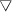, respectively. A further simplification of the Hägg and Nabarro–Frank notations is the Zhdanov (1945) notation, which simply lists in parentheses the series of the sums of the numbers of successive like operators, together with a subscript indicating the repetitions if this is the case. The utility of this compact notation is that, because it directly provides the number of consecutive layers without rotation of 180° in the stacking sequence, it is indicative of the zigzag' chain.

Finally, another appropriate manner of designating the SiC polytypes is the Wyckoff–Jagodzinski notation (Wyckoff, 1948; Jagodzinski, 1949), which examines the ABC stacking sequence and labels each individual layer depending on whether it has cubic or hexagonal local symmetry. Thus, when the layers situated immediately on either side of a given layer are similar the sandwiched layer is denoted as h owing to its local hexagonality, and, conversely, if they are different it is denoted as c owing to its local cubicity (although sometimes k is used instead of c, from the German kubisch).

### 4. The most common SiC polytypes

As mentioned above, the number of SiC polytypes discovered to date is already enormous. Some of them have been observed only sporadically, while others appear regularly. Indeed, there seem to be four polytypes of short stacking sequences (and therefore of small unit cells) that are far more abundant than the rest of the polytypes and can consequently be considered the `basic' SiC structures. In what follows, the crystallographic aspects of these four basic SiC polytypes will be described in more detail.

The sequence T1T2T3 is the only one that can be generated by stacking tetrahedron layers with the same relative orientation and results in the only SiC polytype with cubic symmetry (i.e. the polytype 3C). For this reason this polytype receives the special name of β-SiC. The rest of the polytypes, with hexagonal and rhombohedral symmetries, are all known collectively as α-SiC. Other interesting sequences are T1T2T1T3′ and T1T3T2T1T2T3, which form the two principal SiC polytypes with hexagonal symmetry (i.e. the polytypes 4H and 6H). Finally, it is also important to highlight the sequence T1T2T1T3T2T3T1T3T2T1T2T3T2T1T3′, which yields a typical SiC polytype with rhombohedral symmetry (i.e. the 15R polytype). Shown in Fig. 8are the stacking sequences of tetrahedron layers corresponding to these four basic SiC polytypes, and Table 1lists the various equivalent manners of denoting them.

 Table 1Notations used to designate the SiC polytypes
Tetrahedron layers Ramsdell ABC Hägg Nabarro–Frank Zhdanov Wyckoff–Jagodzinski Type
T1T2T3 3C ABC +++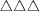c or k β
T1T2T1T3 4H ABAC ++−−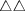22 (ch)2 or (kh)2 α
T1T3T2T1T2T3 6H ACBABC +++−−−33 (hcc)2 or (hkk)2 α
T1T2T1T3T2T3T1T3T2T1T2T3T2T1T3 15R ABACBCACBABCBAC (++−−−)3 ()3 (23)3 (cchch)3 or (kkhkh)3 α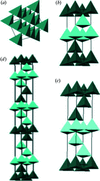Figure 8 Stacking sequences of tetrahedron layers for the four most commonly occurring SiC polytypes: (a) T1T2T3, (b) T1T2T1′T3′, (c) T1T3′T2′T1′T2T3 and (d) T1T2T1′T3′T2′T3T1T3′T2′T1′T2T3T2′T1′T3′. Unit cells not drawn to scale.

The 3C (β-SiC) polytype crystallizes in the cubic system, with a sphalerite-type crystal structure as is shown in Fig. 9(a). The lattice parameter is a0 = b0 = c0 = 4.359 Å, and the space group is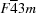.1 Like the diamond structure, β-SiC has a face-centred cubic (f.c.c.) lattice but where the asymmetric unit is constituted by one Si atom placed at the fractional coordinates (0, 0, 0) and one C atom at (,,). The f.c.c. cell contains a total of eight atoms (four of Si and four of C), so that the density is 3.216 Mg m−3.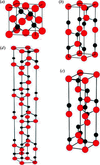Figure 9 Crystal structures for the four most commonly occurring SiC polytypes: (a) 3C, (b) 4H, (c) 6H and (d) 15R. Unit cells not drawn to scale. Si atoms in grey (red in the electronic version of the journal) and C atoms in black.

The 4H polytype crystallizes in the hexagonal system, with a wurtzite-type crystal structure, as is shown in Fig. 9(b). The lattice parameters are a0 = b0 = 3.073 , c0 = 10.053 Å, and the space group is P63mc.2 The asymmetric unit is formed by two Si atoms located at (0, 0, 0) and (,,), as well as two C atoms at (0, 0, 0.1875) and (,, 0.4375). The primitive hexagonal cell contains a total of eight atoms (four of Si and four of C), resulting in a density of 3.239 Mg m−3.

The 6H polytype also crystallizes in the hexagonal system, as is shown in Fig. 9(c). It also has the same type of crystal structure (i.e., wurtzite) and space group (i.e. P63mc) as the 4H polytype. The lattice parameters a0 = b0 and c0 are, however, 3.073 and 15.079 Å, respectively, and the asymmetric unit consists of three Si atoms situated at (0,0,0), (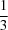,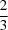,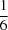) and (,,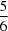), as well as of three C atoms at (0,0,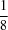), (,, 0.29167) and (,, 0.95833). This time the primitive hexagonal cell contains a total of 12 atoms (six of Si and six of C), giving a density of 3.213 Mg m−3.

Finally, the 15R polytype crystallizes in the rhombohedral (or trigonal) system, with the space group being R3m.3 The lattice parameters of the hexagonal cell (whose volume is three times that of the primitive cell) are a0 = b0 = 3.073, c0 = 37.700 Å. This is shown in Fig. 9(d). The asymmetric unit is given by five Si atoms positioned at (0, 0, 0), (0, 0, 0.1333), (0, 0, 0.4), (0, 0, 0.6) and (0, 0, 0.8667), as well as five C atoms at (0, 0, 0.05), (0, 0, 0.1833), (0, 0, 0.45), (0, 0, 0.65) and (0, 0, 0.9167). Thus, the hexagonal cell contains a total of 30 atoms (15 of Si, and 15 of C), with the density being 3.220 Mg m−3.

As can be seen, these α-SiC polytypes all have the same lattice parameter at the base level, i.e. a0 = b0 = 3.073 Å, but differ essentially in the lattice parameter c0. Nevertheless, the step height along the c axis is the same in all cases (i.e. 10.053/4 = 15.079/6 = 37.70/15).

### 5. Summary

We have presented a worked example of polytypism conceived to help undergraduates in the learning and instructors in the teaching of a crystallography topic that often is not treated in sufficient depth in class. With that educational objective in mind we have chosen the model case of SiC, which exhibits one of the most prolific cases of polytypism known to date. We have begun with a definition of the concept of polytypism, followed by a unified description of the polytypism phenomenon in SiC that is at the same time appealing and useful for those students just initiated into crystallography because it uses only simple arguments of topological constraints to explain the existence of its numerous polytypes and how they develop. Next have we described the typical notations (Ramsdell, ABC, Hägg, Nabarro–Frank, Zhdanov and Wyckoff–Jagodzinski notations) used to designate polytypic variants, and have applied them to the case of the four basic SiC polytypes. Finally we have concluded by discussing in detail the crystal structure of these four polytypes. We believe that this worked example of polytypism may contribute in the tough task of teaching crystallography to noncrystallographers.

### Footnotes

1Or Td2 in the Schönflies notation. The space group number is 216, and the Pearson symbol is cF8. The object being repeated has point group symmetry 43m. The Laue class is m3m. Parallel to the a, b and c crystallographic axes are fourfold symmetry axes of rotary inversion. Parallel to the directions corresponding to the four body diagonals of the cube are threefold symmetry axes. Perpendicular to the directions corresponding to the six lines joining the midpoints of opposite edges of the cube are mirror planes.

2Or C6v4 in the Schönflies notation. The space group number is 186, and the Pearson symbols are hP8 and hP12 for the 4H and 6H polytypes, respectively. The object being repeated has point group symmetry 6mm. The Laue class is 6/mmm. Parallel to the c crystallographic axis is a sixfold screw axis with a rotation of 60° counterclockwise and a unit of translationc0 in the direction parallel to the c crystallographic axis. Perpendicular to the a1, a2 and a3 symmetry axes are mirror planes. Perpendicular to the directions 30° to a1, a2 and a3 and 90° to the c crystallographic axis are c axial glide planes with a unit of translationc0 in the direction parallel to the c crystallographic axis.

3Or C3v5 in the Schönflies notation. The space group number is 160, and the Pearson symbol is hR10. The object being repeated has point group symmetry 3m. The Laue class is 3m. Parallel to the direction trisecting the a, b and c crystallographic axes is a threefold symmetry axis. The object's threefold symmetry axis is parallel to this threefold symmetry axis. Perpendicular to the direction 90° from the direction trisecting the a, b and c crystallographic axes and parallel to the directions halfway between the individual a, b and c crystallographic axes are mirror planes.

### Acknowledgements

This work was supported by the Ministerio de Ciencia y Tecnología (Government of Spain) under grant No. MAT 2010-16848 and by the Junta de Extremadura (Spain) under grants No. GR08071 and No. GR10045.

### ReferencesJOURNAL OFAPPLIEDCRYSTALLOGRAPHY